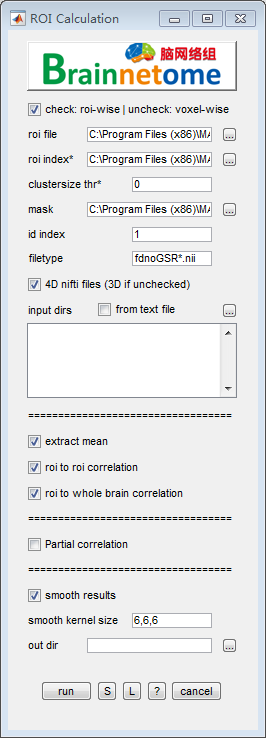# ROI Calculation¶

With a predefined atlas-like ROI file and a descriptive number-label table, the current function can extract mean time series from ROIs and voxels, and calculate Pearson’s correlation as well as its Fisher-z transform. An option is provided to calculate partial correlation between each pair of ROIs, with mean signals of other ROIs as covariates.• roi file: ROIs in one nifti file

• roi index(*): optional. labels of tagged ROIs in a `*.csv` file. For example:

```1,SFG
2,MFG
3,IFG
```
• clustersize thr: threshold of cluster size.

• id index: identifier to find unique string for each subject

• filetype: files in the filetype will be searched in input directories.

• 4D nifti files: if the input data is 4D, check this item. Otherwise uncheck.

• input dirs: directories can be input either using a `*.txt` file or spm select window.

• extract mean: extract mean time series for each ROI

• roi to roi correlation: calculate correlation between pairs of ROI

• roi to whole brain correlation: calculate correlation between each ROI’s mean time series and voxels in the mask.

• Partial correlation: (check to use Partial correlation, uncheck to use Pearson’s correlation) when calculating correlation, between one roi mean time series and voxels/other time series, the rest of roi mean time serieses will be regressed out from the calculation.

• out dir: output directory for saving results.

• Buttons:
• S: Save parameters of the current panel to a `*.mat` file. The `*.mat` can be further loaded for the panel or be used in a script processing.
• L: Load parameters from `*.mat` for the current panel.
• ?: Help information.